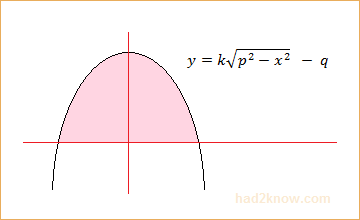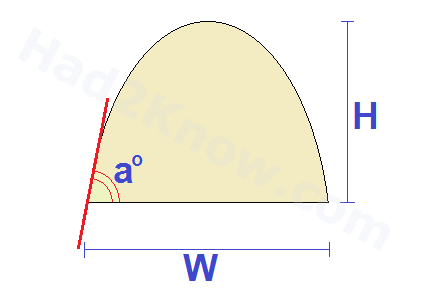# Elliptical Arch Calculator

In architecture, many arches are segments of ellipses. If you know the width of the base of the arch (W), the height from the base to the top of the arch (H), and the angle of the arch's side at the base (a), you can fit the equation of an ellipse to these parameters.

The ellipse equation will have the form y = k⋅sqrt(p² - x²) - q, where k, p, and q depend upon W, H, and a. You can use the calculator below to find equations of elliptical arches.

 Arch Base Width W = Arch Height H = Angle at Base a = o ( 0 < a ≤ 90)### Example 1

An arch is 10 meters wide at the base and 11 meters tall. The angle between the curve of the arch and the base is 90°. If the arch is assumed to be a symmetric arc of an ellipse, what is its equation?

Since the base angle is 90°, i.e., a vertical tangent line, the arch is exactly the top half of an ellipse centered at the base. Since the half-minor axis is 5 meters and the half-major axis is 11 meters, the equation is

y²/121 + x²/25 = 1, or equivalently

y = (11/5)sqrt(25 - x²)

### Example 2

An arch's width is 27 feet and 8 9/16 inches wide; its height is 8 feet. The angle at the base is 60°. If the arch is assumed to be a symmetric arc of an ellipse, what is its equation?

In decimals, the arch has a width of 27.713541666666... feet and a height of 8 feet, for a ratio of 3.464192708333333.... This is very close to 2sqrt(3) = 3.4641016151377544.... Given that special ratio and the fact that the base angle is 60°, the arch is very nearly a circular arc of 120° (one third of a circle). Such a circle has a radius of 16 feet and is centered 8 feet below the base, so its equation is

y = sqrt(256 - x²) - 8

### Example 3

Another arch is 10 meters wide and 11 meters tall, but this arch has an angle of 75° at the base. Attempting to fit these parameters to an ellipse yields no solution, so this arch is not elliptical.

In such cases the arch may be parabolic, hyperbolic, or the arc of some other curve. If the arch is parabolic, the base angle must be exactly arctan(4h/w). If the arch is hyperbolic, the base angle must be strictly less than arctan(4h/w).